Q-Chem 4.3 User’s Manual

# 4.10 Configuration Interaction with Constrained Density Functional Theory (CDFT-CI)

There are some situations in which a system is not well-described by a DFT calculation on a single configuration. For example, transition states are known to be poorly described by most functionals, with the computed barrier being too low. We can, in particular, identify homolytic dissociation of diatomic species as situations where static correlation becomes extremely important. Existing DFT functionals have proved to be very effective in capturing dynamic correlation, but frequently exhibit difficulties in the presence of strong static correlation. Configuration Interaction, well known in wavefunction methods, is a multireference method that is quite well-suited for capturing static correlation; the CDFT-CI technique allows for CI calculations on top of DFT calculations, harnessing both static and dynamic correlation methods.

Constrained DFT is used to compute densities (and Kohn-Sham wavefunctions) for two or more diabatic-like states; these states are then used to build a CI matrix. Diagonalizing this matrix yields energies for the ground and excited states within the configuration space. The coefficients of the initial diabatic states are printed, to show the characteristics of the resultant states.

Since Density-Functional Theory only gives converged densities, not actual wavefunctions, computing the off-diagonal coupling elements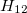is not completely straightforward, as the physical meaning of the Kohn-Sham wavefunction is not entirely clear. We can, however, perform the following manipulation :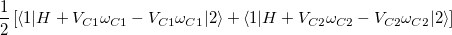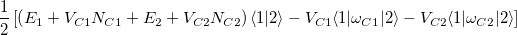(where the converged states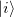are assumed to be the ground state of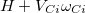with eigenvalue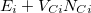). This manipulation eliminates the two-electron integrals from the expression, and experience has shown that the use of Slater determinants of Kohn-Sham orbitals is a reasonable approximation for the quantities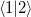and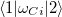.

We note that since these constrained states are eigenfunctions of different Hamiltonians (due to different constraining potentials), they are not orthogonal states, and we must set up our CI matrix as a generalized eigenvalue problem. Symmetric orthogonalization is used by default, though the overlap matrix and Hamiltonian in non-orthogonal basis are also printed at higher print levels so that other orthogonalization schemes can be used after-the-fact. In a limited number of cases, it is possible to find an orthogonal basis for the CDFT-CI Hamiltonian, where a physical interpretation can be assigned to the orthogonal states. In such cases, the matrix representation of the Becke weight operator is diagonalized, and the (orthogonal) eigenstates can be characterized . This matrix is printed as the “CDFT-CI Population Matrix” at increased print levels.

In order to perform a CDFT-CI calculation, the N interacting states must be defined; this is done in a very similar fashion to the specification for CDFT states:

$cdft STATE_1_CONSTRAINT_VALUE_X COEFFICIENT1_X FIRST_ATOM1_X LAST_ATOM1_X TYPE1_X COEFFICIENT2_X FIRST_ATOM2_X LAST_ATOM2_X TYPE2_X ... STATE_1_CONSTRAINT_VALUE_Y COEFFICIENT1_Y FIRST_ATOM1_Y LAST_ATOM1_Y TYPE1_Y COEFFICIENT2_Y FIRST_ATOM2_Y LAST_ATOM2_Y TYPE2_Y ... ... --- STATE_2_CONSTRAINT_VALUE_X COEFFICIENT1_X FIRST_ATOM1_X LAST_ATOM1_X TYPE1_X COEFFICIENT2_X FIRST_ATOM2_X LAST_ATOM2_X TYPE2_X ... STATE_2_CONSTRAINT_VALUE_Y COEFFICIENT1_Y FIRST_ATOM1_Y LAST_ATOM1_Y TYPE1_Y COEFFICIENT2_Y FIRST_ATOM2_Y LAST_ATOM2_Y TYPE2_Y ... ... ...$end


Each state is specified with the CONSTRAINT_VALUE and the corresponding weights on sets of atoms whose average value should be the constraint value. Different states are separated by a single line containing three or more dash characters.

If it is desired to use an unconstrained state as one of the interacting configurations, charge and spin constraints of zero may be applied to the atom range from 0 to 0.

It is MANDATORY to specify a spin constraint corresponding to every charge constraint (and it must be immediately following that charge constraint in the input deck), for reasons described below.

In addition to the $cdft input section of the input file, a CDFT-CI calculation must also set the CDFTCI flag to TRUE for the calculation to run. Note, however, that the CDFT flag is used internally by CDFT-CI, and should not be set in the input deck. The variable CDFTCI_PRINT may also be set manually to control the level of output. The default is 0, which will print the energies and weights (in the diabatic basis) of the N CDFT-CI states. Setting it to 1 or above will also print the CDFT-CI overlap matrix, the CDFT-CI Hamiltonian matrix before the change of basis, and the CDFT-CI Population matrix. Setting it to 2 or above will also print the eigenvectors and eigenvalues of the CDFT-CI Population matrix. Setting it to 3 will produce more output that is only useful during application debugging. For convenience, if CDFTCI_PRINT is not set in the input file, it will be set to the value of SCF_PRINT. As mentioned in the previous section, there is a disparity between our chemical intuition of what charges should be and the actual quantum-mechanical charge. The example was given of LiF, where our intuition gives the lithium atom a formal charge of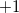; we might similarly imagine performing a CDFT-CI calculation on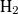, with two ionic states and two spin-constrained states. However, this would result in attempting to force both electrons ofonto the same nucleus, and this calculation is impossible to converge (since by the nature of the Becke weight operators, there will be some non-zero amount of the density that gets proportioned onto the other atom, at moderate internuclear separations). To remedy problems such as this, we have adopted a mechanism by which to convert the formal charges of our chemical intuition into reasonable quantum-mechanical charge constraints. We use the formalism of “promolecule” densities, wherein the molecule is divided into fragments (based on the partitioning of constraint operators), and a DFT calculation is performed on these fragments, completely isolated from each other . (This step is why both spin and charge constraints are required, so that the correct partitioning of electrons for each fragment may be made.) The resulting promolecule densities, converged for the separate fragments, are then added together, and the value of the various weight operators as applied to this new density, is used as a constraint for the actual CDFT calculations on the interacting states. The promolecule density method compensates for the effect of nearby atoms on the actual density that will be constrained. The comments about SCF convergence for CDFT calculations also apply to the calculations used for CDFT-CI, with the addition that if the SCF converges but CDFT does not, it may be necessary to use a denser integration grid or reduce the value of CDFT_THRESH. Analytic gradients are not available. For details on using CDFT-CI to calculate reaction barrier heights, see Ref. Wu:2009. CDFT-CI options are: CDFTCI  Initiates a constrained DFT-configuration interaction calculation TYPE:  LOGICAL DEFAULT:  FALSE OPTIONS:  TRUE Perform a CDFT-CI Calculation FALSE No CDFT-CI RECOMMENDATION:  Set to TRUE if a CDFT-CI calculation is desired. CDFTCI_PRINT  Controls level of output from CDFT-CI procedure to Q-Chem output file. TYPE:  INTEGER DEFAULT:  0 OPTIONS:  0 Only print energies and coefficients of CDFT-CI final states 1 Level 0 plus CDFT-CI overlap, Hamiltonian, and population matrices 2 Level 1 plus eigenvectors and eigenvalues of the CDFT-CI population matrix 3 Level 2 plus promolecule orbital coefficients and energies RECOMMENDATION:  Level 3 is primarily for program debugging; levels 1 and 2 may be useful for analyzing the coupling elements CDFT_LAMBDA_MODE  Allows CDFT potentials to be specified directly, instead of being determined as Lagrange multipliers. TYPE:  BOOLEAN DEFAULT:  FALSE OPTIONS:  FALSE Standard CDFT calculations are used. TRUE Instead of specifying target charge and spin constraints, use the values from the input deck as the value of the Becke weight potential RECOMMENDATION:  Should usually be set to FALSE. Setting to TRUE can be useful to scan over different strengths of charge or spin localization, as convergence properties are improved compared to regular CDFT(-CI) calculations. CDFTCI_SKIP_PROMOLECULES  Skips promolecule calculations and allows fractional charge and spin constraints to be specified directly. TYPE:  BOOLEAN DEFAULT:  FALSE OPTIONS:  FALSE Standard CDFT-CI calculation is performed. TRUE Use the given charge/spin constraints directly, with no promolecule calculations. RECOMMENDATION:  Setting to TRUE can be useful for scanning over constraint values. Note: CDFT_LAMBDA_MODE and CDFTCI_SKIP_PROMOLECULES are mutually incompatible. CDFTCI_SVD_THRESH  By default, a symmetric orthogonalization is performed on the CDFT-CI matrix before diagonalization. If the CDFT-CI overlap matrix is nearly singular (i.e., some of the diabatic states are nearly degenerate), then this orthogonalization can lead to numerical instability. When computing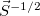, eigenvalues smaller than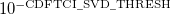are discarded. TYPE:  INTEGER DEFAULT:  4 OPTIONS:for a threshold of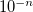. RECOMMENDATION:  Can be decreased if numerical instabilities are encountered in the final diagonalization. CDFTCI_STOP  The CDFT-CI procedure involves performing independent SCF calculations on distinct constrained states. It sometimes occurs that the same convergence parameters are not successful for all of the states of interest, so that a CDFT-CI calculation might converge one of these diabatic states but not the next. This variable allows a user to stop a CDFT-CI calculation after a certain number of states have been converged, with the ability to restart later on the next state, with different convergence options. TYPE:  INTEGER DEFAULT:  0 OPTIONS:stop after converging state(the first state is state)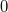do not stop early RECOMMENDATION:  Use this setting if some diabatic states converge but others do not. CDFTCI_RESTART  To be used in conjunction with CDFTCI_STOP, this variable causes CDFT-CI to read already-converged states from disk and begin SCF convergence on later states. Note that the same$cdft section must be used for the stopped calculation and the restarted calculation.

TYPE:
 INTEGER

DEFAULT:
 0

OPTIONS:start calculations on state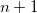RECOMMENDATION:
 Use this setting in conjunction with CDFTCI_STOP.

Many of the CDFT-related rem variables are also applicable to CDFT-CI calculations.

## 4.10.1 C-DFT/CI Examples

Example 4.76  CDFT-CI calculation of couplings between the anionic GFP chromophore (CHR:1-27) and a tyrosine (TYR:28-43) residue. The two diabatic states are Chro-(Ms=0)....Tyr(Ms=0) and Chro(Ms=1/2)....Tyr-(Ms=1/2).

$molecule -1 1 C -1.453000 -1.953000 -0.264000 N -0.278000 -1.402000 -0.440000 N -1.804000 -2.052000 1.091000 C -0.687000 -1.548000 1.806000 O -0.688000 -1.514000 3.031000 C 0.291000 -1.140000 0.799000 C 1.500000 -0.563000 1.254000 H 1.585000 -0.660000 2.346000 C 2.608000 0.030000 0.605000 C 2.763000 0.182000 -0.865000 H 1.926000 -0.073000 -1.543000 C 3.733000 0.548000 1.313000 H 3.682000 0.571000 2.326000 C 3.821000 0.875000 -1.473000 H 3.844000 1.102000 -2.575000 C 4.938000 1.111000 0.700000 H 5.734000 1.441000 1.308000 C 5.037000 1.228000 -0.739000 O 6.011000 1.818000 -1.261000 C -3.000000 -2.533000 1.832000 H -2.859000 -2.250000 2.892000 H -3.829000 -2.121000 1.354000 C -2.373000 -2.282000 -1.448000 H -1.790000 -3.026000 -2.045000 H -2.626000 -1.300000 -1.865000 H -3.054000 -3.631000 1.855000 H -3.308000 -2.854000 -1.357000 C 7.648000 -5.429000 0.303000 H 8.028000 -4.514000 0.845000 H 7.274000 -5.098000 -0.671000 C 6.499001 -5.986000 1.016000 C 6.462999 -6.032001 2.390000 H 7.284000 -5.579000 2.957000 C 5.243000 -6.435000 3.018000 H 5.190001 -6.315001 4.035000 C 4.242001 -7.048000 2.189000 O 3.095000 -7.615000 2.715000 H 2.500999 -7.869000 1.979000 C 5.454000 -6.469000 0.200000 H 5.565001 -6.363000 -0.835000 C 4.294001 -7.003000 0.803000 H 3.469000 -7.324000 0.139000 H 8.511000 -6.108000 0.245000$end

$rem symmetry off sym_ignore = true method = b3lyp basis = cc-pvdz unrestricted = true scf_convergence = 8 max_scf_cycles = 200 cdftci = true cdftci_print = 2 cdft_thresh = 7$end

$cdft 1.0 1.0 1 27 0.0 1.0 1 27 s -------------- 0.0 1.0 1 27 -1.0 1.0 1 27 s$end